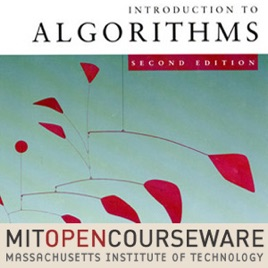23 episodes

This course teaches techniques for the design and analysis of efficient algorithms, emphasizing methods useful in practice. Topics covered include: sorting; search trees, heaps, and hashing; divide-and-conquer; dynamic programming; amortized analysis; graph algorithms; shortest paths; network flow; computational geometry; number-theoretic algorithms; polynomial and matrix calculations; caching; and parallel computing.# Introduction to Algorithms (2005) MIT

• Technology

This course teaches techniques for the design and analysis of efficient algorithms, emphasizing methods useful in practice. Topics covered include: sorting; search trees, heaps, and hashing; divide-and-conquer; dynamic programming; amortized analysis; graph algorithms; shortest paths; network flow; computational geometry; number-theoretic algorithms; polynomial and matrix calculations; caching; and parallel computing.

• video
Lecture 01: Administrivia/Introduction/Analysis of Algorithms, Insertion Sort, Mergesort

## Lecture 01: Administrivia/Introduction/Analysis of Algorithms, Insertion Sort, Mergesort

• 1 hr 20 min
• video
Lecture 02: Asymptotic Notation/Recurrences/Substitution, Master Method

## Lecture 02: Asymptotic Notation/Recurrences/Substitution, Master Method

• 1 hr 10 min
• video
Lecture 03: Divide-and-Conquer: Strassen, Fibonacci, Polynomial Multiplication

## Lecture 03: Divide-and-Conquer: Strassen, Fibonacci, Polynomial Multiplication

• 1 hr 8 min
• video
Lecture 04: Quicksort, Randomized Algorithms

## Lecture 04: Quicksort, Randomized Algorithms

• 1 hr 20 min
• video
Lecture 05: Linear-time Sorting: Lower Bounds, Counting Sort, Radix Sort

## Lecture 05: Linear-time Sorting: Lower Bounds, Counting Sort, Radix Sort

• 1 hr 16 min
• video
Lecture 06: Order Statistics, Median

• 1 hr 8 min

5.0 out of 5
2 Ratings

2 Ratings